Here are a few calculators for electronic/radio circuits. They are crude, but they work.

Note that the inductance unit is nano-Henries (nH) (1uH = 1000nH). Capacitance unit is pico-Farads (pf) and resistance unit is ohms. Decimals are not allowed in the input fields, if you enter a value of 1.9925 then the assumed input value will be 1.

## Resistors In Parallel (or Capacitors in Series)

Formula: Effective Resistance = (R1 x R2) / (R1 + R2)

 R1 R2 Effective Resistance <>

## Reactance of a Capacitor

Formula: Reactance = 1 / (2 * Pi * F * C)

 Freq (Hz) [F] Cap (pF) [C] Reactance <>

## Reactance of an Inductor

Formula: Reactance = 2 * Pi * F * L

 Freq (Hz) [F] Induct (nH) [L] Reactance <>

## Basic frequency

Formula: Frequency = 1 / (2 * Pi * Sqrt(LC))

 Capacitance (pF) [C] Inductance (nH) [L] Frequency

## Single Layer Coil

 Formula: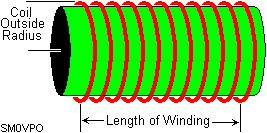Inductance (uH) [L] Length of Winding [l] Coil Outside Radius [r] All sizes in millimeters Turns required [N] <>

## Multi-Layer Coil

 Formula: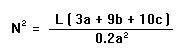Inductance (uH) [L] Winding depth+Form Dia [a] Winding Length [b] Winding Depth [c] Turns required (All sizes in millimeters) <>

## Heatsink Evaluation

Calculates the effective temperature rise coefficient of a measured heatsink. Area is EXPOSED surface area of heatsink in square centimeters.

 Formula: Degrees Centigrade per Watt = 50 / Sqrt(Area sq-cm )

 Area (sq-cm) [A] Exposed areas only. Degrees C / Watt <>

## Heatsink Calculation

Calculates heatsink surface area needed for a given heatsink temperature coefficient.

 Formula: Area Required (sq-cm) = Sq (50/C-watt)

 Tenths of Degrees C-Watt (1 degree = 10) Area Required (sq-cm) <>

## Attenuators - "T" and "H" Type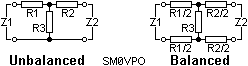Formulas:The Input/Output powers may be in any electrical unit you prefer, eg: Meggawatts, Picowatts, Joules, Hamster-treadmill revolutions per hour etc.

 Enter Input Power Enter Output Power Enter Input Impedance Enter Output Impedance R1 value (for unbalanced) R2 value (for unbalanced) R1/2 value (for balanced) R2/2 value (for balanced) R3 value <>

Negative values of resistance occur when impossible values of input and output impedance and attenuation are entered. For example, it is not possible to drive a 50-ohm line from a 600-ohm line and have a voltage attenuation factor of less than 6.78

## Attenuators - Square (or Box) Type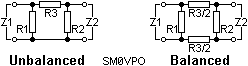Formulas: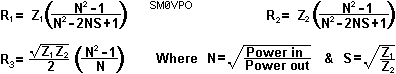The Input/Output powers may be in any electrical unit you prefer, eg: Meggawatts, Picowatts, Joules, Hamster-treadmill revolutions per hour, etc.

 Enter Input Power Enter Output Power Enter Input Impedance Enter Output Impedance R1 value R2 value R3 value (for unbalanced) R3/2 value (for balanced) SM0VPO <>

Again, negative values indicate an impossible attenuation situation.Enter your search terms Submit search form

Ham radio Data Center - free schematics | Free HAM Directory | About me | Acronyms  | CW | Data Sheets | Docs | Download | E-mail | HOME | Ham projects | Hobby circuits | Photo galery | PIC | QTH photos |